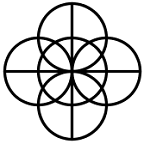### Base Dozen Forum

Would you like to react to this message? Create an account in a few clicks or log in to continue.
Base Dozen Forum

A board for discussion of the number twelve as the base of numeration in mathematics and physics.

### Dozenal Clock

Dozenal Clock
 Local Dozenal Time: Local Decimal Time:

### Latest topics

» Colour ClassificationToday at 6:57 pm by Phaethon

» How a Dozenal Metrological System Should be DevelopedTue Sep 05, 2023 8:33 pm by Phaethon

» Optimal Analogue ClockSun Jul 02, 2023 5:10 pm by Phaethon

» Dozenal Fifths Better than Decimal ThirdsTue Jun 13, 2023 11:00 pm by Phaethon

» Unit Power PrefixesSat May 13, 2023 12:19 pm by Phaethon

» Old School Textbook DuodecimalSun Apr 02, 2023 10:59 pm by Phaethon

» Perpetual CalendarTue Mar 14, 2023 12:54 pm by Phaethon

» PotencyMon Feb 27, 2023 5:48 pm by Phaethon

» MnemonicsTue Feb 07, 2023 5:43 pm by Phaethon

### Top posters

Phaethonwendy.krieger# Factor DensityPhaethon

Posts : 146
Points : 237
Join date : 2019-08-05
Consider that the most important property of a number for it to be used as a base of enumeration and division is the number of factors it has. Under this supposition, a base with more factors will be better than a base that has fewer factors, while other conditions are equal as far as possible. For example, the base thirty should be a better base than the bases twenty-nine or thirty-one, because thirty has more factors than twenty-nine or thirty-one, although the numbers are of similar size. The number of factors, say $$F_B$$, a base number $$B$$ has is dependent on its prime factorisation:
$\prod_{i=1}^{i=n} {p_i}^{a_i}$
where $$p_i$$ is the $$i^{th}$$ prime and $$a_i$$ is its exponent, and can be calculated by the formula:
$F_B = \prod_{i=1}^{n} ({a_i + 1})$
However, there tends to be a cost to gaining more factors in that the size of the base tends to increase such that it becomes less practical. To take into account the size of the base, divide its number of factors by the number of factors a base in the vicinity of its size would be expected to have to form a measure $$D_B$$ of the density of factors:
$D_B = \frac{F_B }{1+\log_2 {B}}$
According to this computed measure, bases that are powers of two have a unitary density of factors and are called efficient, bases that have a factor density less than one have a lower density of factors and are called deficient because they have fewer factors than expected for their size, and bases that have a factor density of more than one have a greater density of factors. A table can be drawn comparing factor densities of various bases:

Table of Base Factor Densities
 Base, decimally $$D_B$$ decimally $$D_B$$ dozenally 2 1 ①⁏ 3 0.7737 ⓪⁏⑨③⑤ 4 1 ①⁏ 6 1.1158 ①⁏①④⑧ 8 1 ①⁏ 9 0.7194 ⓪⁏⑧⑦⑦ 10 0.9255 ⓪⁏⑪①③ 12 1.3086 ①⁏③⑧⑤ 16 1 ①⁏ 18 1.1606 ①⁏①⑪① 24 1.4324 ①⁏⑤②③ 30 1.3544 ①⁏④③⓪ 36 1.4587 ①⁏⑤⑥ 48 1.5186 ①⁏⑥②⑧ 60 1.7374 ①⁏⑧⑩② 72 1.6737 ①⁏⑧①⓪ 120 2.0236 ②⁏⓪③ 180 2.1197 ②⁏①⑤ 210 1.8361 ①⁏⑩⓪ 360 2.5285 ②⁏⑥④① 720 2.8594 ②⁏⑩③⑨ 840 2.9867 ②⁏⑪⑩① 2520 3.9027 ③⁏⑩⑩
As one increases the base, those with higher factor densities than of smaller bases tend to be highly composite numbers. According to the factor density, bases that increase in size without introducing a greater number of factors over smaller bases tend to be worse. Thus, bases eight and ten are worse than base six. Base twelve has a good factor density for its size and there is not a better base until the double dozen. This factor density as a computed measure of base efficiency does not penalise an increase in size of the base enough, as base eight for example has the same density as base 1024 decimally, although most would admit that the latter as a pure base would be far more impractical.Phaethon

Posts : 146
Points : 237
Join date : 2019-08-05
If the factor density be scaled by doubling it, it becomes an indication of how the distribution of factors of the base behaves in comparison to the factors of a binary power. Another computed measure comparing bases to binary by the number of factors is to raise the base, B, to the power of the reciprocal of the subtraction of one from the number of factors, FB, of the base, to produce an efficiency base score. That is:
$B^{\frac{1}{F_B - 1}}$
Bases with a score greater than the number two may be said to be deficient, while bases having a score less than the number two may be called superefficient. The so-called perfect number twenty-eight is slightly superefficient and very similar in its density of factors to binary powers. Decimal is deficient and this is a very bad trait; superefficient vigesimal would be better.

If we double the reciprocal of this efficiency base score, it produces the same rank for the three dozen bases I examined as the original factor density does, except for the bases six and twenty, which are swapped. Thus, there is justification in using this version of factor density instead of the original one in this topic. This version of factor density produces similar values for smaller bases but has the advantage of being less extreme or more tame for large highly composite numbers. I used it to produce a more reasonable optimality score in which impractically large highly composite number bases tend to be ranked not quite so highly.

Reference:
https://dozenal.forumotion.com/t52-base-optimality

Current date/time is Sat Sep 30, 2023 10:59 pm